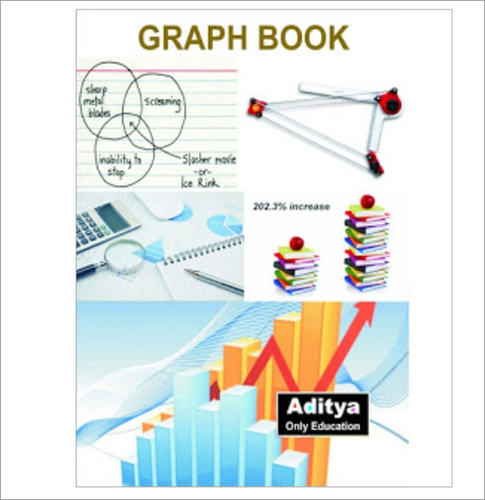0Graph Notebook
Price And Quantity
• 1000
• Piece/Pieces,
• 16 INR
Product Description
We offer graph notebook to the customers. The use of graph papers is to draw graphs in them. Certain classes specifically, mathematics, economics, and science classes require the perfect display of figures, formulae for solving the questions clearly and accurately. The graph paper is the writing paper, printed with grid lines. The graph is perfect for accurately plotting the graphical representation. Graph notebook is often demanded for plotting the data points, solving the mathematical equations, and making dimensions of a sketch. There are some questions or problems in the math book that cannot be solved without the use of graphs. Due to this, the demand of this notebook is rising in the academic sector.
Related Products